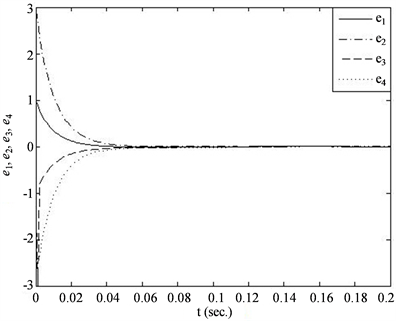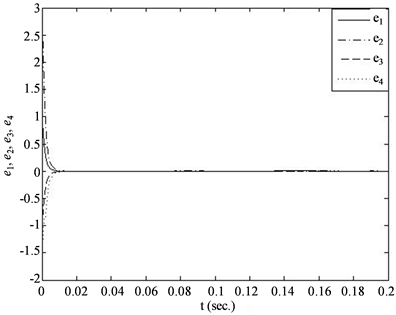# 混沌系统的同步控制Synchronization and Control of Chaotic System

DOI: 10.12677/OJNS.2018.66059, PDF, HTML, XML, 下载: 485  浏览: 1,688

Abstract: Since the applications in the field of secure communication and so on, the synchronization of frac-tional-order chaotic system catches many attentions and is researched extensively. In this paper, we launch of a series of research on chaotic synchronization by the method of combing theory prove and numerical simulations. A robust synchronization scheme of factional-order hyperchaotic systems is promoted. Through theory analysis, we get that the synchronization error can be forced and kept inside a ball around the origin when the drive system and response system both have external noises, and the radius of the ball can be chosen by designing proper controller.

1. 引言

1963年数学家Lorenz发表了题为“确定性的非周期流”的文章  ，刊登在著名的《大气科学杂志》上。基于对流问题的研究，Lorenz提出了一个可以描述天气变化情况的常微分方程组，得出了初始值的极其微小的变化也可以引起天气十分巨大变化的结论。于是著名的“蝴蝶效应”就诞生了：“一只蝴蝶在巴西轻轻地拍动几下翅膀，有可能在三个月后引起美国得克萨斯州的的一场龙卷风”。由于首次提出了该理论，Lorenz被称为“混沌学之父”。

2. 混沌的定义

2.1. Li-Yorke的混沌定义

1975年发表在美国《数学月刊》的一篇题为“周期3蕴含混沌”的论文，第一次提出了“混沌”一词并给出了其数学表示形式，该混沌定义被称为Li-Yorke定义 。

$F:\left[a,b\right]×R\to \left[a,b\right]$ , $\left(x,\lambda \right)↦F\left(x,\lambda \right)$ , $x\in R$ . (1)

${x}_{n+1}=F\left({x}_{n},\lambda \right)$ , ${x}_{n}\in \left[a,b\right]$ . (2)

1) 存在一切周期的周期点；

2) 存在不可数子集 $S\subset \left[a,b\right]$ ，使得

$\underset{n\to \infty }{\mathrm{lim}}\mathrm{inf}|{F}^{n}\left(x,\lambda \right)-{F}^{n}\left(y,\lambda \right)|=0$ , $x,y\in S$ , $x\ne y$ , (3)

$\underset{n\to \infty }{\mathrm{lim}}\mathrm{sup}|{F}^{n}\left(x,\lambda \right)-{F}^{n}\left(y,\lambda \right)|>0$ , $x,y\in S$ , $x\ne y$ , (4)

$\underset{n\to \infty }{\mathrm{lim}}\mathrm{sup}|{F}^{n}\left(x,\lambda \right)-{F}^{n}\left(p,\lambda \right)|>0$$x\in S$ ，p为周期点。 (5)

2.2. Devaney的混沌定义

1) f对初始条件具有敏感依赖性。对任意 $x\in V$$\sigma >0$ ，在x的领域中存在y及自然数n，使得 $d\left({f}^{n}\left(x\right),{f}^{n}\left(y\right)\right)>\sigma$

2) f是拓扑传递的。对 $V$ 上的任意开集X和Y，存在 $k>0$ ，使得 ${f}^{k}\left(X\right)\cap Y\ne \Phi$

3) f的周期点集在 $V$ 中稠密。

3. 混沌系统同步概述

1990年，Ott和York在发表的《混沌控制》一文中提出了混沌控制的参数扰动方法，即OGY方法，将混沌系统的运动成功地镇定在不稳定的周期轨道上。1990年Pecora和Carroll提出混沌同步的概念，并将其成功应用于保密通信。混沌同步从本质上来讲是一种广义的混沌控制，是指使两个混沌系统的运动轨道逐渐趋于一致并保持稳定。混沌同步的类型主要有：完全同步、投影同步、相同步、延迟同步等。目前，许多新的混沌同步方法不断地被提出，如脉冲同步法、函数投影同步、鲁棒同步、观测器同步法等。

3.1. 混沌同步方法

3.1.1. 鲁棒同步

$\frac{{\text{d}}^{q}x}{\text{d}{t}^{q}}=F\left(x\right)$ , (6)

$\frac{{\text{d}}^{q}y}{\text{d}{t}^{q}}=G\left(y\right)+U$ , (7)

3.1.2. 脉冲同步

$\stackrel{˙}{x}=f\left(t,x\right)$ , (8)

$f:{R}_{+}×{R}^{n}\to {R}^{n}$ 是连续函数， $x\in {R}^{n}$ 代表状态变量。

$\left\{\begin{array}{l}\stackrel{˙}{y}=f\left(t,y\right),\text{}t\ne {t}_{k}\\ \Delta y=y\left({t}_{k}^{+}\right)-y\left({t}_{k}^{-}\right)={B}_{k}e,\text{}\left(t={t}_{k}\text{,}k=1,2,\cdots \right)\\ y\left({t}_{0}^{+}\right)={y}_{0}\end{array}$ (9)

${B}_{k}$$n×n$ 阶矩阵， $e={\left[{e}_{1},{e}_{2},\cdots ,{e}_{n}\right]}^{\text{T}}={\left[{y}_{1}-{x}_{1},{y}_{2}-{x}_{2},\cdots ,{y}_{n}-{x}_{n}\right]}^{\text{T}}$ 。定义一个不连续的变量集 $\left\{{t}_{k}\right\}$ ，满足如下条件： ${t}_{1}<{t}_{2}<\cdots <{t}_{k}<{t}_{k+1}<\cdots$ ，当 $k\to \infty$ 时，有 ${t}_{k}\to \infty$${t}_{k}\left(k=1,2,\cdots \right)$ 是驱动系统传递给响应

$\left\{\begin{array}{l}\stackrel{˙}{e}=f\left(t,y\right)-f\left(t,x\right),\text{}t\ne {t}_{k}\\ \Delta e={B}_{k}e,\text{}t={t}_{k}\end{array}$ . (10)

3.1.3. 投影同步

$\stackrel{˙}{x}=F\left(x\right)$ , (11)

$\stackrel{˙}{y}=F\left(y\right)+U$ , (12)

$\underset{t\to \infty }{\mathrm{lim}}‖y-\alpha x‖=0$ (13)

$\underset{t\to \infty }{\mathrm{lim}}‖y-x‖=0$ , (14)

$\underset{t\to \infty }{\mathrm{lim}}‖y+x‖=0$ , (15)

3.1.4. 广义同步

$\stackrel{˙}{x}=F\left(x\right)$ , (16)

$\stackrel{˙}{y}=G\left(x,y\right)$ , (17)

$\underset{t\to \infty }{\mathrm{lim}}‖y\left(t\right)-\varphi \left(x\left(t\right)\right)‖=0$ (18)

4. 具有扰动的分数阶超混沌系统的异结构鲁棒同步

4.1. 鲁棒同步方案的设计

$\frac{{\text{d}}^{q}x}{\text{d}{t}^{q}}=F\left(x\right)+{D}_{1}\left(t\right)$ , (19)

$\frac{{\text{d}}^{q}y}{\text{d}{t}^{q}}=G\left(y\right)+U+{D}_{2}\left(t\right)$ , (20)

(20)我们可以得到误差动力系统

 , (21)

$U=\beta e-C$ , (22)

$\frac{{\text{d}}^{q}e}{\text{d}{t}^{q}}=\left(-\alpha +B\right)e+D$ . (23)

$|{d}_{j}\left(t\right)|<\stackrel{˜}{d}=2d$ , (24)

$D=Pe$ , (25)

$\frac{{\text{d}}^{q}e}{\text{d}{t}^{q}}=Ee=\left(-\alpha +Β+P\right)e$ , (26)

$|{e}_{j}\left(t\right)|<{R}_{j}$ , (27)

$|{p}_{j}\left(t\right)|<\stackrel{˜}{d}/{R}_{j}$ , (28)

${\alpha }_{i}>\stackrel{˜}{d}/{R}_{j}+{b}_{i}$ (29)

4.2. 数值仿真

4.2.1. 分数阶超混沌系统的鲁棒同步

$\left\{\begin{array}{l}\frac{{\text{d}}^{\alpha }{x}_{1}}{\text{d}{t}^{\alpha }}=a\left({x}_{2}-{x}_{1}\right)+{x}_{4}+{d}_{11}\\ \frac{{\text{d}}^{\alpha }{x}_{2}}{\text{d}{t}^{\alpha }}=c{x}_{1}-{x}_{2}-{x}_{1}{x}_{3}+{d}_{12}\\ \frac{{\text{d}}^{\alpha }{x}_{3}}{\text{d}{t}^{\alpha }}={x}_{1}{x}_{2}-b{x}_{3}+{d}_{13}\\ \frac{{\text{d}}^{\alpha }{x}_{4}}{\text{d}{t}^{\alpha }}=-{x}_{2}{x}_{3}+r{x}_{4}+{d}_{14}\end{array}$ , (30)

${D}_{2}\left(t\right)=\left({d}_{21},{d}_{22},{d}_{23},{d}_{24}\right)$ 得到系统的表示形式如下：

$\left\{\begin{array}{l}\frac{{\text{d}}^{\alpha }{y}_{1}}{\text{d}{t}^{\alpha }}=l\left({y}_{2}-{y}_{1}\right)+{u}_{1}+{d}_{21}\\ \frac{{\text{d}}^{\alpha }{y}_{2}}{\text{d}{t}^{\alpha }}=m{y}_{1}+n{y}_{2}-{y}_{1}{y}_{3}-{y}_{4}+{u}_{2}+{d}_{22}\\ \frac{{\text{d}}^{\alpha }{y}_{3}}{\text{d}{t}^{\alpha }}={y}_{1}{y}_{2}-p{y}_{3}+{u}_{3}+{d}_{23}\\ \frac{{\text{d}}^{\alpha }{y}_{4}}{\text{d}{t}^{\alpha }}={y}_{1}+g+{u}_{4}+{d}_{24}\end{array}$ , (31), (32)

$\left\{\begin{array}{l}{u}_{1}=-{\alpha }_{1}e{}_{1}-a{e}_{2}-e{}_{4}+\left(l-a\right){y}_{1}-\left(l-a\right){y}_{2}+{y}_{4}\\ {u}_{2}=-{\alpha }_{2}{e}_{2}+\left(-c+{x}_{3}\right){e}_{1}+{y}_{1}{e}_{3}+\left(-m+c\right){y}_{1}-\left(n+1\right){y}_{2}+{y}_{4}\\ {u}_{3}=-{\alpha }_{3}{e}_{3}-{x}_{2}{e}_{1}-{y}_{1}{e}_{2}-\left(b-p\right){y}_{3}\\ {u}_{4}=-{\alpha }_{4}{e}_{4}-{y}_{1}+r{y}_{4}-{x}_{2}{x}_{3}-g\end{array}$ , (33)

$\left\{\begin{array}{l}\frac{{\text{d}}^{\alpha }{e}_{1}}{\text{d}{t}^{\alpha }}=\left(-{\alpha }_{1}-a\right)e{}_{1}+{d}_{1}\\ \frac{{\text{d}}^{\alpha }{e}_{2}}{\text{d}{t}^{\alpha }}=\left(-{\alpha }_{2}-1\right){e}_{2}+{d}_{2}\\ \frac{{\text{d}}^{\alpha }{e}_{3}}{\text{d}{t}^{\alpha }}=\left(-{\alpha }_{3}-b\right){e}_{3}+{d}_{3}\\ \frac{{\text{d}}^{\alpha }{e}_{4}}{\text{d}{t}^{\alpha }}=\left(-{\alpha }_{4}+r\right){e}_{4}+{d}_{4}\end{array}$ . (34)

$E=\left[\begin{array}{cccc}-{\alpha }_{1}-a+{p}_{1}\left(t\right)& 0& 0& 0\\ 0& -{\alpha }_{2}-1+{p}_{2}\left(t\right)& 0& 0\\ 0& 0& -{\alpha }_{3}-b+{p}_{3}\left(t\right)& 0\\ 0& 0& 0& -{\alpha }_{4}+r+{p}_{4}\left(t\right)\end{array}\right]$ , (35)

$\left\{\begin{array}{l}{\alpha }_{1}>\stackrel{˜}{d}/{R}_{1}-a\\ {\alpha }_{2}>\stackrel{˜}{d}/{R}_{2}-1\\ {\alpha }_{3}>\stackrel{˜}{d}/{R}_{3}-b\\ {\alpha }_{4}>\stackrel{˜}{d}/{R}_{4}+r\end{array}$ . (36)Figure 1. Robust synchronization errors of system (30) and system (31) when ${R}_{i}=0.05$

${R}_{i}=0.01$ ，那么由式(36)可知当选取 ${\alpha }_{1}={\alpha }_{2}={\alpha }_{3}={\alpha }_{4}=400$ 时符合鲁棒同步的条件。当选取状态变量初值为状态变量的初值为 $\left({x}_{1}\left(0\right),{x}_{2}\left(0\right),{x}_{3}\left(0\right),{x}_{4}\left(0\right)\right)=\left(2,1,3,4\right)$$\left({y}_{1}\left(0\right),{y}_{2}\left(0\right),{y}_{3}\left(0\right),{y}_{4}\left(0\right)\right)=\left(3,4,2,1\right)$ ，此时分数阶超混沌系统(30)和(31)获得鲁棒同步。同步结果如图2所示。Figure 2. Robust synchronization errors of system (30) and system (31) when ${R}_{i}=0.01$

5. 结论

  Lorenz, E.N. (1963) Deterministic Nonperodic Flow. Journal of the Atmospheric Sciences, 20, 130-141. https://doi.org/10.1175/1520-0469(1963)020<0130:DNF>2.0.CO;2  郝柏林. 分岔, 混沌, 奇怪吸引子、湍流及其它[J]. 物理学进展, 1983, 3(3): 329-426.  Slotine, J.J. and Li, W.P. (1991) Applied Nonlinear Control. Prentice Hall, USA.  Chen, G. and Dong, X. (1998) From Chaos to Order: Methodplpgies, Perspectives and Applications. World Scientific, Singapore. https://doi.org/10.1142/3033  王光瑞, 于熙龄, 陈世刚. 混沌的控制、同步与应用[M]. 北京: 国防工业出版社, 2001.  王兴元. 复杂非线性系统中的混沌[M]. 北京: 电子工业出版社, 2003.  Hubler, A. and Lüscher, E. (1989) Reso-nant Stimulation and Control of Nonlinear Oscillators. Naturwissenschaften, 76, 67-69. https://doi.org/10.1007/BF00396707  Ott, E., Grebogi, C. and Yorke, J.A. (1990) Controlling Chaos. Physical Review Letters, 64, 1196-1199. https://doi.org/10.1103/PhysRevLett.64.1196  Pecora, L.M. and Carroll, T.L. (1990) Synchronization in Chaotic Systems. Physical Review Letters, 64, 821-827. https://doi.org/10.1103/PhysRevLett.64.821  Bai, E.W., Lonngren, K.E. and Sprott, J.C. (2002) On the Synchronization of a Class of Electronic Circuits that Exhibit Chaos. Chaos, Solitons & Fractals, 13, 1515-1521. https://doi.org/10.1016/S0960-0779(01)00160-6  Sun, J.T. and Zhang, Y.P. (2003) Stability Analysis of Impulsive Control Systems. IEE Proceedings Control Theory and Applications, 150, 331-334. https://doi.org/10.1049/ip-cta:20030599  Guan, X.P, Fan, Z.P., Chen, C.L., et al. (2002) Chaotic Control and Its Application on Secure Communication. National Defence Industry Press, Beijing.  Feki, M. (2003) An Adaptive Chaos Synchronization Scheme Applied to Secure Communication. Chaos, Solitons & Fractals, 18, 141-148. https://doi.org/10.1016/S0960-0779(02)00585-4  Wang, Y., Guan, Z.H. and Wang, H.O. (2003) Feedback and Adaptive control for the Synchronization of Chen System via a Single Variable. Physics Letters A, 312, 34-40. https://doi.org/10.1016/S0375-9601(03)00573-5  Wang, Y.W., Guan, Z.H. and Xiao, J.W. (2004) Impulsive Synchronization for a Class of Continuous Systems. Chaos, 14, 199-203. https://doi.org/10.1063/1.1644351  Asheghan, M.M., Hamidi Beheshti, M.T. and Tavazoei, M.S. (2011) Robust Synchronization of Perturbed Chen’s Fractional-Order Chaotic Systems. Commu-nications in Nonlinear Science and Numerical Simulation, 16, 1044-1051. https://doi.org/10.1016/j.cnsns.2010.05.024  Li, T.Y. and Yorke, J.A. (1975) Period Three Implies Chaos. American Mathematical Monthly, 82, 982-992.